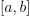# module `onnx_tools.model_checker`#

## Short summary#

module `mlprodict.onnx_tools.model_checker`

Investigate issues happening with float32.

source on GitHub

## Classes#

class

truncated documentation

`MissingInputError`

Raised when an input is missing.

## Functions#

function

truncated documentation

`astype_range`

Computes ranges for every number in an array once converted into float32. The function returns two matrices which …

`check_onnx`

Checks consistency of the model.

`enumerate_random_inputs`

Enumerates random matrices.

`onnx_shaker`

Shakes a model ONNX. Explores the ranges for every prediction. Uses `astype_range()`

## Documentation#

Investigate issues happening with float32.

source on GitHub

exception mlprodict.onnx_tools.model_checker.MissingInputError#

Bases: `RuntimeError`

Raised when an input is missing.

mlprodict.onnx_tools.model_checker.astype_range(arr, dtype=<class 'numpy.float32'>, force=1)#

Computes ranges for every number in an array once converted into float32. The function returns two matrices which produces two numbers a et b, the number rounded to float32 is in interval.

Parameters:
• arr – array

• dtype – type to convert to

• force – does something like [i] +/- force |i - [i]|

Returns:

minimum, maximum

source on GitHub

mlprodict.onnx_tools.model_checker.check_onnx(model, use_onnx=False, known_results=None, path=None)#

Checks consistency of the model.

Parameters:
• model – onnx graph

• use_onnx – calls onnx.checker.check_model

• known_results – known results

• path – path to a node (through subgraphs)

source on GitHub

mlprodict.onnx_tools.model_checker.enumerate_random_inputs(inputs, n=100, dtype=<class 'numpy.float32'>, force=1)#

Enumerates random matrices.

Parameters:
• inputs – inputs (dictionary)

• n – number of iterations

• dtype – type to convert to

• force – does something like [i] +/- force |i - [i]|

source on GitHub

mlprodict.onnx_tools.model_checker.onnx_shaker(oinf, inputs, output_fct, n=100, dtype=<class 'numpy.float32'>, force=1)#

Shakes a model ONNX. Explores the ranges for every prediction. Uses `astype_range`

Parameters:
• oinf – object of type `OnnxInference`

• inputs – inputs

• output_fct – output function which extracts a single array from the output

• dtype – type to convert to

• force – does something like [i] +/- force |i - [i]|

Returns:

ranges for each predictions

See notebook Precision loss due to float32 conversion with ONNX for an example of use.

source on GitHub

mlprodict.onnx_tools.model_checker.randint(low, high=None, size=None, dtype=int)#

Return random integers from low (inclusive) to high (exclusive).

Return random integers from the “discrete uniform” distribution of the specified dtype in the “half-open” interval [low, high). If high is None (the default), then results are from [0, low).

Note

New code should use the `integers` method of a `default_rng()` instance instead; please see the Quick Start.

### Parameters#

lowint or array-like of ints

Lowest (signed) integers to be drawn from the distribution (unless `high=None`, in which case this parameter is one above the highest such integer).

highint or array-like of ints, optional

If provided, one above the largest (signed) integer to be drawn from the distribution (see above for behavior if `high=None`). If array-like, must contain integer values

sizeint or tuple of ints, optional

Output shape. If the given shape is, e.g., `(m, n, k)`, then `m * n * k` samples are drawn. Default is None, in which case a single value is returned.

dtypedtype, optional

Desired dtype of the result. Byteorder must be native. The default value is int.

New in version 1.11.0.

### Returns#

outint or ndarray of ints

size-shaped array of random integers from the appropriate distribution, or a single such random int if size not provided.

random_integerssimilar to randint, only for the closed

interval [low, high], and 1 is the lowest value if high is omitted.

random.Generator.integers: which should be used for new code.

### Examples#

```>>> np.random.randint(2, size=10)
array([1, 0, 0, 0, 1, 1, 0, 0, 1, 0]) # random
>>> np.random.randint(1, size=10)
array([0, 0, 0, 0, 0, 0, 0, 0, 0, 0])
```

Generate a 2 x 4 array of ints between 0 and 4, inclusive:

```>>> np.random.randint(5, size=(2, 4))
array([[4, 0, 2, 1], # random
[3, 2, 2, 0]])
```

Generate a 1 x 3 array with 3 different upper bounds

```>>> np.random.randint(1, [3, 5, 10])
array([2, 2, 9]) # random
```

Generate a 1 by 3 array with 3 different lower bounds

```>>> np.random.randint([1, 5, 7], 10)
array([9, 8, 7]) # random
```

Generate a 2 by 4 array using broadcasting with dtype of uint8

```>>> np.random.randint([1, 3, 5, 7], [, ], dtype=np.uint8)
array([[ 8,  6,  9,  7], # random
[ 1, 16,  9, 12]], dtype=uint8)
```